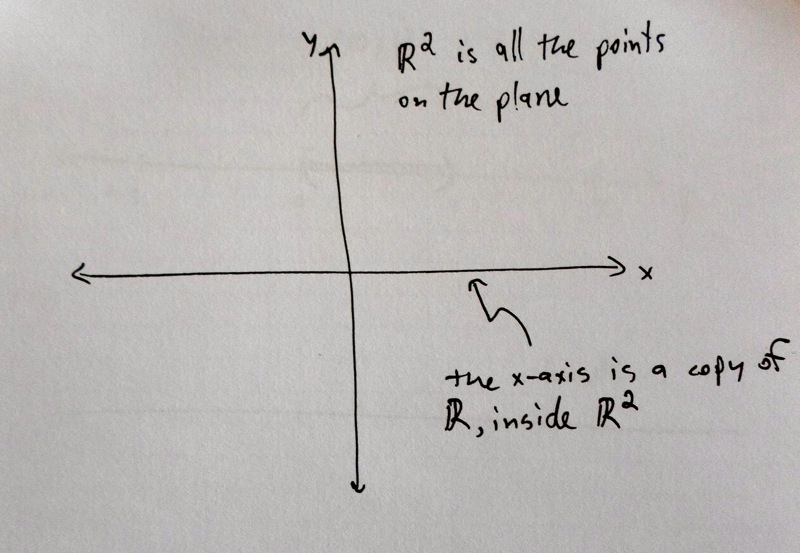# In-Depth

Here are more careful details about why $$|\mathbb{R}^4| = |\mathbb{R}|$$.

Rather than focus on $$|\mathbb{R}^4| = |\mathbb{R}|$$, we will in fact show that $$|\mathbb{R}^n| = |\mathbb{R}|$$ for any positive integer $$n$$. As in the short explanation, we will prove the (notationally) simpler case of $$|\mathbb{R}^2| = |\mathbb{R}|$$, and from the proof it will be clear how to do the case of general $$n$$.

A key tool is the Cantor-Bernstein-Schroeder theorem. This theorem says that if an infinite set $$A$$ can be put into one-to-one correspondence with a subset $$B$$ of a set $$C$$, then it makes sense to say $$| A| ≤ | C|$$. And furthermore, if we can show $$| C| ≤ | A|$$, then we can conclude that $$| A| = | C|$$.

In other words, these sizes of infinities, which aren't really numbers, behave like numbers in this sense: $$a ≤ b$$ and $$b ≤ a$$ implies $$a = b$$. We will use this theorem without proving it; the proof is surprisingly involved.

Our first step towards showing $$|\mathbb{R}^2| = |\mathbb{R}|$$ will be to put $$\mathbb{R}$$ into one-to-one correspondence with a subset of $$\mathbb{R}^2$$. Remember that $$\mathbb{R}$$ is the continuous one-dimensional real line, and $$\mathbb{R}^2$$ is the continuous two-dimensional plane.

We can imagine a copy of $$\mathbb{R}$$ sitting inside $$\mathbb{R}^2$$, as the $$x$$-axis of a coordinate system. The elements of $$\mathbb{R}^2$$ are of the form $$[x, y]$$, where $$x$$ and $$y$$ are real numbers. Then the $$x$$-axis is all the points of the form $$[x, 0]$$, where $$x$$ is any real number. (The $$x$$-axis is exactly the points where the $$y$$-coordinate is zero.)So we can construct a one-to-one correspondence between $$\mathbb{R}$$ and the $$x$$-axis of the plane. Every real number $$x$$ gets assigned to $$[x, 0]$$. This clearly hits every point on the $$x$$-axis once and only once. Since the $$x$$-axis is a subset of the whole plane $$\mathbb{R}^2$$, we can use the Cantor-Bernstein-Schroeder theorem to conclude that $$|\mathbb{R}| ≤ |\mathbb{R}^2|$$.

Next we will put $$\mathbb{R}^2$$ into one-to-one correspondence with a subset of $$\mathbb{R}$$. But here we have to be a little careful. Every element of $$\mathbb{R}^2$$ can be written as $$[x, y]$$, but for many elements this is not unique. For example,

$1 = 0.999999...$

So the element $$[1, 4]$$ can be written as any of the following.

$[1, 4]\\ [0.99999…, 4]\\ [1, 3.999999…]\\ [0.9999…, 3.9999…]$

If we naively took each point $$[x,y]$$ and assigned it to the real number created by interweaving the digits of $$x$$ and $$y$$, as discussed in the short explanation, then we get into trouble. The same point $$[1, 4]$$ would get assigned to four different real numbers, depending on which representation we chose!

$14\\ 04.9090909090…\\ 13.0909090909…\\ 03.9999999….$

These are all very different real numbers.

To avoid this problem, we can declare which to choose. Let's choose the first. Now our assignment rule from $$\mathbb{R}^2$$ to $$\mathbb{R}$$ is: for each element $$[x, y]$$, write it in such a way that there are no endlessly-repeated nines. Then (after lining up the decimals and adding zeros to the left and right as necessary, so that both $$x$$ and $$y$$ have the same number of digits), interweave the digits of $$x$$ and $$y$$ to form one new real number.

Now this assignment rule is well-defined. And for every real number that gets hit by this assignment rule, it is possible to unweave the digits, to recover the element $$[x, y]$$ that hit it. In other words, this assignment rule makes a one-to-one correspondence between $$\mathbb{R}^2$$ and the set of real numbers hit by the assignment rule.

But we still have one problem: not every real number gets hit by the assignment rule. For example, consider the real number

$04.90909090…$

If we unweave this real number, we get

$[0.999999… , 4.00000].$

But this is the same as $$[1, 4]$$, and according to our assignment rule this goes to $$14$$. In other words, there is no element $$[x,y]$$ of $$\mathbb{R}^2$$ that gets assigned to $$4.909090…$$.

Fortunately this isn't a big problem. What we have shown is that there is a one-to-one correspondence between $$\mathbb{R}^2$$ and a subset of $$\mathbb{R}$$ (the subset of real numbers that do get hit by our assignment rule). Using the Cantor-Bernstein-Schroeder theorem we mentioned above, this is enough to conclude that $$|\mathbb{R}^2| ≤ |\mathbb{R}|$$. And since we already showed that $$|\mathbb{R}| ≤ |\mathbb{R}^2|$$, we have proved that $$|\mathbb{R}^2| = |\mathbb{R}|$$.

To prove that $$|\mathbb{R}^4| = |\mathbb{R}|$$, we would just have to alter the argument above to do a four-fold weaving. For example,

$[1.01, 4.6, 0.8, 13]$

would get assigned to

$00011403.06801 = 11403.06801.$

To prove that $$|\mathbb{R}^n| = |\mathbb{R}|$$ for any positive integer $$n$$, we would do an $$n$$-fold weaving.

Top